# JP6361171B2 - Equivalent circuit - Google Patents

## Info

Publication number
JP6361171B2
JP6361171B2 JP2014042726A JP2014042726A JP6361171B2 JP 6361171 B2 JP6361171 B2 JP 6361171B2 JP 2014042726 A JP2014042726 A JP 2014042726A JP 2014042726 A JP2014042726 A JP 2014042726A JP 6361171 B2 JP6361171 B2 JP 6361171B2
Authority
JP
Japan
Prior art keywords
circuit
internal electrode
terminal
equivalent circuit
inductance
Prior art date
Legal status (The legal status is an assumption and is not a legal conclusion. Google has not performed a legal analysis and makes no representation as to the accuracy of the status listed.)
Active
Application number
JP2014042726A
Other languages
Japanese (ja)
Other versions
JP2015170004A (en
Inventor

Original Assignee
Tdk株式会社
Ｔｄｋ株式会社
Priority date (The priority date is an assumption and is not a legal conclusion. Google has not performed a legal analysis and makes no representation as to the accuracy of the date listed.)
Filing date
Publication date
Application filed by Tdk株式会社, Ｔｄｋ株式会社 filed Critical Tdk株式会社
Priority to JP2014042726A priority Critical patent/JP6361171B2/en
Publication of JP2015170004A publication Critical patent/JP2015170004A/en
Application granted granted Critical
Publication of JP6361171B2 publication Critical patent/JP6361171B2/en
Active legal-status Critical Current
Anticipated expiration legal-status Critical

• 239000003990 capacitor Substances 0 claims description 85
• 238000004088 simulation Methods 0 claims description 23
• 230000000694 effects Effects 0 description 6

## Images

•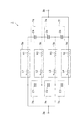•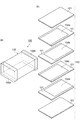•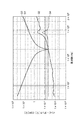•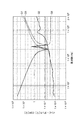•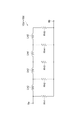•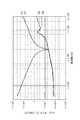•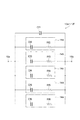••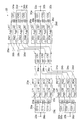••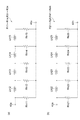•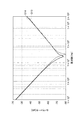•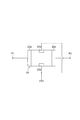•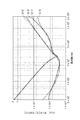•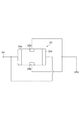## Description

The present invention relates to an equivalent circuit for simulation of a multilayer capacitor.

As a prior art in this type of field, for example, one described in Patent Document 1 is known. In the equivalent circuit model of the multilayer chip capacitor described in Patent Document 1, the equivalent series capacitance and part of the equivalent series resistance of the basic equivalent circuit are generated near the corners and ends of the internal electrodes in the multilayer chip capacitor. Capacitance and resistance are provided as circuit elements related to the abnormal characteristics of the electromagnetic field distribution. With this configuration, the equivalent circuit model suppresses the occurrence of an error from the actual circuit performance.

JP 2012-3409 A

As described above, the equivalent circuit is required to suppress the difference from the characteristics of the actual multilayer capacitor and to obtain characteristics that approximate the characteristics of the actual multilayer capacitor.

An object of the present invention is to provide an equivalent circuit capable of obtaining characteristics approximating those of an actual multilayer capacitor.

An equivalent circuit according to the present invention is an equivalent circuit for simulating a multilayer capacitor, and includes a first circuit portion indicating an internal electrode of the multilayer capacitor, a second circuit portion indicating a capacitance of the multilayer capacitor, There are three or more first circuit parts, and at least two of the first circuit parts have inductance components, and the second circuit part includes one first circuit part and another first circuit. And a mutual inductance is set between the inductance components of the first circuit unit.

This equivalent circuit is provided with three or more first circuit portions indicating internal electrodes. Thus, in the equivalent circuit, by providing three or more first circuit portions, an internal resonance phenomenon occurs at a predetermined frequency. At this time, a current (resonant current) larger than usual flows through each first circuit unit. When the resonance current flows through the first circuit portion having a loss, it appears as a loss peak in the impedance characteristics of the entire equivalent circuit. This peak occurs in an actual multilayer capacitor. Therefore, the equivalent circuit can be close to the characteristics of the actual multilayer capacitor. In the equivalent circuit, a mutual inductance is set between the inductance components. Thereby, in the equivalent circuit, a physical phenomenon (an imbalance of current between the internal electrodes or a resonance phenomenon generated between the internal electrodes) that occurs in the actual multilayer capacitor can be realized. Therefore, in the equivalent circuit, it is possible to obtain characteristics that approximate the characteristics of the actual multilayer capacitor.

In one embodiment, the first circuit unit has a resistance component, and the resistance component has a plurality of inductance elements connected in series and one end connected to each end of each inductance element, and in parallel with each other. And a plurality of connected resistance elements. Thus, by configuring the resistance component of the first circuit portion with an inductance element and a resistance element, it is possible to consider the skin effect, and the resistance component (ESR: equivalent series resistance) in the high frequency band is increased. Therefore, it is possible to obtain characteristics that further approximate the characteristics of the actual multilayer capacitor.

In one embodiment, the second circuit unit includes a first capacitor element and a series circuit in which the second capacitor element and the resistor element are connected in series, and one or more series circuits are provided. The first capacitor element and the series circuit may be connected in parallel. By configuring the second circuit portion with the first capacitive element and the series circuit in this way, it is possible to consider dielectric loss, and the capacitance component on the low frequency side can be increased and the resistance component can be decreased. Therefore, it is possible to obtain characteristics that further approximate the characteristics of the actual multilayer capacitor.

In one embodiment, it has the 1st-the 4th terminal, the 1st terminal and 3 terminals are electrically connected by the 1st circuit part, and the 2nd terminal and 4 terminals are the 1st terminal and The first circuit unit that is different from the first circuit unit connected to the third terminal may be electrically connected. With this configuration, the equivalent circuit is configured as a feedthrough capacitor. Here, in the feedthrough capacitor, when the second terminal electrode and the fourth terminal electrode electrically connected by the internal electrode among the four terminal electrodes are connected to the ground, the second terminal electrode and the fourth terminal electrode are connected to the ground. Noise currents of the same size and in opposite directions flow toward the terminal electrodes. At this time, the magnetic flux generated by the current flowing toward the second terminal electrode and the magnetic flux generated by the current flowing toward the fourth terminal electrode cancel each other. Thereby, in the feedthrough capacitor, ESL (equivalent series inductance) can be reduced. The equivalent circuit models the structure of the feedthrough capacitor and sets the mutual inductance between the inductance components. Thereby, in the equivalent circuit, characteristics equivalent to those of the feedthrough capacitor can be obtained, and the effect of canceling the magnetic flux in the feedthrough capacitor can be modeled.

According to the present invention, it is possible to obtain characteristics that approximate the characteristics of the actual multilayer capacitor.

It is a figure which shows the equivalent circuit which concerns on 1st Embodiment. It is a figure which shows the structure of the multilayer capacitor | condenser which has an equivalent circuit shown in FIG. It is a figure which shows the simulation result of the frequency characteristic of the impedance and ESR in the equivalent circuit shown in FIG. It is a figure which shows the simulation result of the frequency characteristic of the impedance and ESR in the equivalent circuit of a comparative example. It is a circuit diagram which shows the structure of the resistance part of the internal electrode part in the equivalent circuit which concerns on 2nd Embodiment. It is a figure which shows the simulation result of the frequency characteristic of the impedance and ESR in an equivalent circuit provided with the resistance part shown in FIG. It is a circuit diagram which shows the structure of the electrostatic capacitance circuit of the electrostatic capacitance part in the equivalent circuit which concerns on 3rd Embodiment. It is a figure which shows the simulation result of the frequency characteristic of the impedance and ESR in the equivalent circuit provided with the electrostatic capacitance circuit shown in FIG. It is a figure which shows the equivalent circuit which concerns on 4th Embodiment. It is a figure which shows the structure of the feedthrough capacitor which has an equivalent circuit shown in FIG. It is a figure which shows the structure of a resistive element. It is a figure which shows the simulation result of the frequency characteristic of S parameter of the equivalent circuit shown in FIG. It is a figure which shows the measurement circuit of S parameter. It is a figure which shows the simulation result of the frequency characteristic of the impedance and ESR in the equivalent circuit shown in FIG. It is a figure which shows the measurement circuit of an impedance and ESR.

Hereinafter, preferred embodiments of the present invention will be described in detail with reference to the accompanying drawings. In the description of the drawings, the same or equivalent elements will be denoted by the same reference numerals, and redundant description will be omitted.

[First Embodiment]
FIG. 1 is a diagram illustrating an equivalent circuit according to the first embodiment. An equivalent circuit 1 shown in FIG. 1 is, for example, a circuit used for circuit design or analysis simulation using electronic components, and is applied to a circuit design (analysis) program executed on a computer.

As shown in FIG. 1, the equivalent circuit 1 includes a first terminal 3a and a second terminal 3b, internal electrode portions (first circuit portions) 5a to 5d, and capacitance portions (second circuit portions) 7a to 7f. And. As shown in FIG. 2, the equivalent circuit 1 shown in FIG. 1 includes an element body 102 in which a dielectric layer 101 is laminated, and two layers of first internal electrodes 104a, 104c, and 2 arranged in the element body 102. A layered second internal electrode 104b, 104d, a first terminal electrode 106a connected to the first internal electrode 104a, 104c, and a second terminal electrode 106b connected to the second internal electrode 104b, 104d. 3 is an equivalent circuit of the type capacitor 100. The first internal electrodes 104a and 104c and the second internal electrodes 104b and 104d are alternately arranged. The first terminal 3a corresponds to the first terminal electrode 106a, and the second terminal 3b corresponds to the second terminal electrode 106b. The internal electrode portions 5a to 5d correspond to the first internal electrodes 104a and 104c and the second internal electrodes 104b and 104d.

The internal electrode portions 5 a to 5 d indicate the internal electrodes 104 a to 104 d of the multilayer capacitor 100. Four internal electrode portions 5a to 5d are provided. This indicates that in the multilayer capacitor 100, four layers of internal electrodes 104a to 104d are arranged. The internal electrode portions 5a and 5c are connected to the first terminal 3a. That is, the internal electrode portions 5a and 5c correspond to the first internal electrodes 104a and 104c. The internal electrode portions 5b and 5d are connected to the second terminal 3b. That is, the internal electrode portions 5b and 5d correspond to the second internal electrodes 104b and 104d.

The internal electrode portion 5a includes an inductance element (inductance component) L1 and a resistance element R1. The inductance element L1 and the resistance element R1 are connected in series to form an RL series circuit. The inductance element L1 represents parasitic inductance due to internal electrodes, connection conductors, and the like. The resistance element R1 represents a conductor loss such as an internal electrode or a connection conductor.

The internal electrode portion 5b has an inductance element L2 and a resistance element R2. The internal electrode portion 5c has an inductance element L3 and a resistance element R3. The internal electrode portion 5d has an inductance element L4 and a resistance element R4.

Capacitance portions 7 a to 7 f indicate the capacitance of the multilayer capacitor 100. Capacitance parts 7a-7f are arranged between internal electrode parts 5a-5d. Specifically, the capacitance part 7a and the capacitance part 7d are arranged between the internal electrode part 5a and the internal electrode part 5b. That is, the electrostatic capacitance parts 7a and 7d represent the electrostatic capacity generated by the internal electrode parts 5a and 5b.

Similarly, the electrostatic capacitance part 7b and the electrostatic capacitance part 7e are arrange | positioned between the internal electrode part 5b and the internal electrode part 5c. That is, the electrostatic capacitance parts 7b and 7e represent the electrostatic capacity generated by the internal electrode parts 5b and 5c. Between the internal electrode part 5c and the internal electrode part 5d, the electrostatic capacitance part 7c and the electrostatic capacitance part 7f are arranged. That is, the electrostatic capacitance parts 7c and 7f represent the electrostatic capacity generated by the internal electrode parts 5c and 5d. Each capacitance part 7a-7f has capacitance elements C1, C2, C3, C4, C5, and C6, respectively.

In the present embodiment, mutual inductance is set between the inductance elements L1 to L4 in the internal electrode portions 5a to 5d. Specifically, the coupling coefficient K1 between the inductance element L1 and the inductance element L2, the coupling coefficient K2 between the inductance element L1 and the inductance element L3, the coupling coefficient K3 between the inductance element L1 and the inductance element L4, the inductance element L2 and the inductance element L3. Are set, a coupling coefficient K5 between the inductance element L2 and the inductance element L4, and a coupling coefficient K6 between the inductance element L3 and the inductance element L4. Each of the coupling coefficients K1 to K6 is appropriately set according to the characteristics of the actual multilayer capacitor.

FIG. 3 is a diagram showing a simulation result of frequency characteristics of impedance and ESR in the equivalent circuit shown in FIG. FIG. 4 is a diagram showing simulation results of impedance and ESR frequency characteristics in the equivalent circuit of the comparative example. 3 and 4, the vertical axis represents impedance [Ω] and ESR (equivalent series resistance) [Ω], and the horizontal axis represents frequency [Hz]. 3 and 4, the measured impedance value of the actual multilayer capacitor is indicated by G1, the measured value of ESR is indicated by G2, the impedance in the equivalent circuit 1 of this embodiment is indicated by G3, G5, and the ESR is indicated by G4. This is indicated by G6.

As shown in FIG. 3, the actual multilayer capacitor has a minimum impedance at a frequency of around 200 MHz, as shown by a graph G1. This is because the reactance due to the capacitance of the capacitor and the inductance due to the equivalent series inductance cancel each other, and the total reactance value becomes zero. In the actual multilayer capacitor, as shown in the graph G2, an ESR peak occurs at a frequency near 800 MHz. This is due to internal resonance. On the other hand, in the equivalent circuit 1, as shown in the graph G3, the impedance is a minimum value near the frequency of 200 MHz, and as shown in the graph G4, an ESR peak occurs near the frequency of 800 MHz. ing. That is, in the equivalent circuit 1, it is possible to obtain characteristics substantially equivalent to the actually measured values of the actual multilayer capacitor.

On the other hand, as shown in FIG. 4, in a circuit in which mutual inductance is not set between the inductance elements L1 to L4 (coupling coefficients K1 to K6 are set to 0), as shown in graphs G5 and G6, the actual product This shows characteristics different from those of the multilayer capacitor (graphs G1 and G2). In the equivalent circuit of the comparative example, the internal resonance phenomenon that occurs in the actual multilayer capacitor is not properly reproduced, and thus exhibits different characteristics from the actual multilayer capacitor. Therefore, the effectiveness of setting the mutual inductance (coupling coefficients K1 to K6) between the inductance elements L1 to L4 of the equivalent circuit 1 was confirmed.

As described above, the equivalent circuit 1 according to the present embodiment includes the internal electrode portions 5a to 5d. As described above, in the equivalent circuit 1, by providing three or more internal electrode portions (internal electrodes) (four in this embodiment), an internal resonance phenomenon occurs at a predetermined frequency. At this time, a current (resonant current) larger than normal flows through each of the internal electrode portions 5a to 5d. When the resonance current flows through the internal electrode having a loss, it appears as a loss peak in the impedance characteristic of the entire equivalent circuit 1. Therefore, as shown in FIG. 2, the equivalent circuit 1 can be close to the characteristics of an actual multilayer capacitor.

In the equivalent circuit 1 of the present embodiment, a mutual inductance is set between the inductance elements L1 to L4. As a result, in the equivalent circuit 1, a physical phenomenon (an imbalance of current between the internal electrodes or a resonance phenomenon generated between the internal electrodes) that occurs in the actual multilayer capacitor can be realized. Therefore, the equivalent circuit 1 can obtain characteristics that approximate the characteristics of the actual multilayer capacitor.

[Second Embodiment]
Next, the second embodiment will be described. The equivalent circuit according to the second embodiment is different from the first embodiment in that it includes resistance portions (resistance components) 10a to 10d instead of the resistance elements R1 to R4 of the internal electrode portions 5a to 5d. FIG. 5 is a circuit diagram showing a configuration of the resistance portion of the internal electrode portion in the equivalent circuit according to the second embodiment.

As shown in FIG. 5, the resistance portion (resistance component) 10 a includes inductance elements Lh2 to Lh5 and resistance elements Rh1 to Rh5. The inductance elements Lh2, Lh3, Lh4, and Lh5 are connected in series in this order. One end of the inductance element Lh2 is connected to the terminal 9a. In this embodiment, four inductance elements Lh2 to Lh5 and five resistance elements Rh1 to Rh5 are used. However, the number of inductance elements and resistance elements may be appropriately set according to the design.

One end of the resistance element Rh1 is connected to one end (terminal 9a) of the inductance element Lh2, and the other end is connected to the terminal 9b. The resistor element Rh2 has one end connected to the other end of the inductance element Lh2 and one end of the inductance element Lh3, and the other end connected to the terminal 9b. That is, one end of the resistance element Rh1 and the resistance element Rh2 is connected to both ends of the inductance element Lh2. The resistance element Rh3 has one end connected to the other end of the inductance element Lh3 and one end of the inductance element Lh4, and the other end connected to the terminal 9b. The resistance element Rh4 has one end connected to the other end of the inductance element Lh4 and one end of the inductance element Lh5, and the other end connected to the terminal 9b. The resistor element Rh5 has one end connected to the other end of the inductance element Lh5 and the other end connected to the terminal 9b. Two inductance elements and one resistance element form a T-type circuit. The resistance portions 10b to 10d also have the same configuration as that of the resistance portion 10a.

FIG. 6 is a diagram illustrating a simulation result of the impedance and ESR frequency characteristics in the equivalent circuit including the resistance unit illustrated in FIG. 5. In FIG. 6, the vertical axis represents impedance [Ω] and ESR (equivalent series resistance) [Ω], and the horizontal axis represents frequency [Hz]. In FIG. 6, the measured impedance value of the actual multilayer capacitor is indicated by G1, the measured value of ESR is indicated by G2, the impedance in the equivalent circuit of this embodiment is indicated by G7, and the ESR is indicated by G8.

As shown in FIG. 6, in the equivalent circuit including the resistance portions 10 a to 10 d in the internal electrode portions 5 a to 5 d, as shown by the graphs G <b> 7 and G <b> 8, the actual multilayer capacitor of the actual product is used in a band of 1 GHz or less. Characteristics substantially equivalent to the actually measured values (graphs G1 and G2) are obtained, and the ESR peak generated at a frequency of about 800 MHz is also modeled. Regarding the fact that there is a difference from the actually measured value in the frequency band of 1 GHz or higher, the actual multilayer capacitor has a large number of internal electrodes, and high-order resonance occurs between the internal electrodes. In the equivalent circuit of this embodiment, since the internal electrode portions 5a to 5d are provided with four layers of internal electrodes, only the primary resonance is modeled. Therefore, by increasing the number of internal electrode portions in the equivalent circuit of the present embodiment, it is possible to approach an actual measurement value even in a frequency band of 1 GHz or higher.

As described above, in the equivalent circuit according to the second embodiment, the resistance units 10a to 10d are configured by the inductance elements Lh2 to Lh5 and the resistance elements Rh1 to Rh5 connected in series. In actual multilayer capacitors, the higher the frequency, the higher the resistance of the conductor due to the skin effect. In the present embodiment, the resistance portions 10a to 10d are configured by the inductance elements Lh2 to Lh5 and the resistance elements Rh1 to Rh5 in order to realize the loss generated in the internal electrode in more detail. Thereby, an equivalent circuit in consideration of the skin effect can be configured. Therefore, the resistance component at a high frequency is increased, and the characteristics of the actual multilayer capacitor can be further approximated.

[Third Embodiment]
Subsequently, the third embodiment will be described. The equivalent circuit according to the third embodiment is different from the first embodiment in that capacitance circuits 12a to 12f are provided instead of the capacitance elements C1 to C6 of the capacitance units 7a to 7f. In this equivalent circuit, the configuration of the internal electrode portions 5a to 5d is the same as that of the second embodiment. That is, the internal electrode portions 5a to 5d include resistance portions 10a to 10d. FIG. 7 is a circuit diagram showing the configuration of the capacitance circuit of the capacitance section of the equivalent circuit according to the third embodiment.

As shown in FIG. 7, the capacitance circuits 12a to 12f include a capacitance element (first capacitance element) Cf1 and CR series circuits 14a to 14e. In the CR series circuit 14a, a capacitance element (second capacitance element) Cf2 and a resistance element Rf2 are connected in series. Similarly, in the CR series circuits 14b to 14e, capacitance elements Cf3 to Cf6 and resistance elements Rf3 to Rf6 are connected in series. One or more CR series circuits 14a to 14e are provided, and five CR series circuits are provided in the present embodiment. The number of CR series circuits may be set as appropriate according to the design.

The capacitance element Cf1 and the CR series circuits 14a to 14e are connected in parallel. Specifically, the capacitance element Cf1 has one end connected to the terminal 15a and the other end connected to the terminal 15b. In the CR series circuit 14a, one end of the capacitance element Cf2 is connected to the terminal 15a, and the other end of the resistance element Rf2 is connected to the terminal 15b. The same applies to the CR series circuits 14b to 14e.

FIG. 8 is a diagram illustrating simulation results of impedance and ESR frequency characteristics in an equivalent circuit including the capacitance circuit illustrated in FIG. In FIG. 8, the vertical axis represents impedance [Ω] and ESR (equivalent series resistance) [Ω], and the horizontal axis represents frequency [Hz]. In FIG. 8, the measured impedance value of the actual multilayer capacitor is indicated by G9, the measured value of ESR is indicated by G10, the impedance in the equivalent circuit of this embodiment is indicated by G11, and the ESR is indicated by G12.

As shown in FIG. 8, in the equivalent circuit including the capacitance circuits 12a to 12f, as shown by the graphs G11 and G12, in the band below the frequency of 1 GHz, the actual measurement value (graph) Characteristics substantially equivalent to those of G9, G10) are obtained. In the actual measurement value, an ESR peak occurs near 20 MHz, but in this equivalent circuit, an ESR peak occurs near 20 MHz. Further, in the frequency band of 1 MHz or less, the actual multilayer capacitor has a characteristic that the ESR is inversely proportional to the frequency due to dielectric loss, but this equivalent circuit is similar to the actual multilayer capacitor. Characteristics are obtained.

As described above, in the equivalent circuit according to the third embodiment, the capacitance circuits 12a to 12f of the capacitance units 7a to 7f have the capacitance element Cf1 and the CR series circuits 14a to 14e connected in parallel. It has a configuration. By configuring the capacitance circuits 12a to 12f in this way, dielectric loss can be taken into consideration, and the capacitance component on the low frequency side can be increased and the resistance component can be decreased. Further, in this equivalent circuit, the resistance portions 10a to 10d are provided in order to realize the loss generated in the internal electrode in more detail. Therefore, in this equivalent circuit, since the loss due to the skin effect generated in the internal electrode and the influence of the dielectric loss caused by the dielectric are taken into account, the characteristics of the actual multilayer capacitor can be modeled in more detail. .

[Fourth Embodiment]
Subsequently, a fourth embodiment will be described. FIG. 9 is a diagram illustrating an equivalent circuit according to the fourth embodiment. As shown in FIG. 9, the equivalent circuit 20 includes a first terminal 22a, a second terminal 22b, a third terminal 22c and a fourth terminal 22d, internal electrode portions 23a to 23d, internal electrode portions 24a to 24d, and internal electrode portions. 26a to 26d and internal electrode portions 28a to 28d, capacitance portions 29a to 29c, capacitance portions 30a to 30c, capacitance portions 32a to 32c, capacitance portions 34a to 34c, and capacitance portions 36a to 36a 36c.

As shown in FIG. 10, the equivalent circuit 20 shown in FIG. 9 has a rectangular parallelepiped shape with a dielectric layer 201 laminated thereon, a pair of end faces 202a and 202b opposed in the longitudinal direction, and a pair opposed in the width direction. Element body 202 having side surfaces 202c and 202d and a pair of main surfaces 202e and 202f facing each other in the height direction, first to fourth internal electrodes 204a to 204d disposed in the element body 202, and first internal electrodes 204a and the third internal electrode 204c and connected to the first terminal electrode 206a and the third terminal electrode 206c respectively disposed on the end faces 202a and 202b of the element body 202, and to the second internal electrode 204b and the fourth internal electrode 204d. And a second terminal electrode 206b and a fourth terminal electrode 206d disposed on the side surfaces 202c and 202d of the element body 202, respectively. It is an equivalent circuit of the capacitors 200. The first terminal 22a corresponds to the first terminal electrode 206a, and the second terminal 22b corresponds to the second terminal electrode 206b. The third terminal 22c corresponds to the third terminal electrode 206c, and the fourth terminal 22d corresponds to the fourth terminal electrode 206d.

In the feedthrough capacitor 200, the first terminal electrode 206a and the third terminal electrode 206c are conducted through the first internal electrode 204a and the third internal electrode 204c, and the second terminal electrode 206b and the fourth terminal electrode 206d are connected. Conductivity is established via the second internal electrode 204b and the fourth internal electrode 204d, and the capacitance between the first terminal electrode 206a and the third terminal electrode 206c and the second terminal electrode 206b and the fourth terminal electrode 206d. have. The feedthrough capacitor 200 is used by passing a signal between the first terminal electrode 206a and the third terminal electrode 206c, and connecting the second terminal electrode 206b and the fourth terminal electrode 206d to the ground of the circuit. Thereby, in the feedthrough capacitor 200, high frequency noise included in the signal component passing through the first terminal electrode 206a and the third terminal electrode 206c flows to the ground via the second terminal electrode 206b and the fourth terminal electrode 206d. High frequency noise passing through the first terminal electrode 206a and the third terminal electrode 206c can be removed.

The internal electrode portions 23 a to 23 d, the internal electrode portions 24 a to 24 d, the internal electrode portions 26 a to 26 d, and the internal electrode portions 28 a to 28 d indicate the internal electrodes 204 a to 204 d of the feedthrough capacitor 200. Four internal electrode portions 23a to 23d, internal electrode portions 24a to 24d, internal electrode portions 26a to 26d, and internal electrode portions 28a to 28d are provided. This indicates that in the feedthrough capacitor 200, four layers of internal electrodes 204a to 204d are arranged.

The internal electrode portion 23a, the internal electrode portion 24a, the internal electrode portion 26a, and the internal electrode portion 28a correspond to the first internal electrode 204a. The internal electrode portion 23a and the internal electrode portion 26a are connected to the first terminal 22a and the third terminal 22c. The internal electrode portion 23b, the internal electrode portion 24b, the internal electrode portion 26b, and the internal electrode portion 28b correspond to the second internal electrode 204b. The internal electrode portion 24b and the internal electrode portion 28b are connected to the second terminal 22b and the fourth terminal 22d.

The internal electrode portion 23c, the internal electrode portion 24c, the internal electrode portion 26c, and the internal electrode portion 28c correspond to the third internal electrode 204c. The internal electrode portion 23c and the internal electrode portion 26c are connected to the first terminal 22a and the third terminal 22c. The internal electrode portion 23d, the internal electrode portion 24d, the internal electrode portion 26d, and the internal electrode portion 28d correspond to the fourth internal electrode 204d. The internal electrode portion 24d and the internal electrode portion 28d are connected to the second terminal 22b and the fourth terminal 22d.

The internal electrode portion 23a includes an inductance element L11 and a resistance portion R11. The inductance element L11 and the resistance unit R11 are connected in series to form an RL series circuit. The internal electrode portion 23b has an inductance element L12 and a resistance portion R12. The internal electrode portion 23c has an inductance element L13 and a resistance portion R13. The internal electrode portion 23d has an inductance element L14 and a resistance portion R14.

The internal electrode portion 24a includes an inductance element L21 and a resistance portion R21. The inductance element L21 and the resistance unit R21 are connected in series to form an RL series circuit. The internal electrode portion 24b includes an inductance element L22 and a resistance portion R22. The internal electrode portion 24c has an inductance element L23 and a resistance portion R23. The internal electrode portion 24d has an inductance element L24 and a resistance portion R24.

The internal electrode portion 26a includes an inductance element L31 and a resistance portion R31. The inductance element L31 and the resistor portion R31 are connected in series to form an RL series circuit. The internal electrode portion 26b has an inductance element L32 and a resistance portion R32. The internal electrode portion 26c includes an inductance element L33 and a resistance portion R33. The internal electrode portion 26d includes an inductance element L34 and a resistance portion R34.

The internal electrode portion 28a includes an inductance element L41 and a resistance portion R41. The inductance element L41 and the resistance unit R41 are connected in series to form an RL series circuit. The internal electrode portion 28b has an inductance element L42 and a resistance portion R42. The internal electrode portion 28c includes an inductance element L43 and a resistance portion R43. The internal electrode portion 28d has an inductance element L44 and a resistance portion R44.

As shown to Fig.11 (a), each of resistance part R11-R14, R31-R34 is provided with resistance element Rh11-Rh15 and inductance element Lh12-Lh15. The inductance elements Lh12, Lh13, Lh14, and Lh15 are connected in series in this order. One end of the inductance element Lh12 is connected to the terminal 40a.

One end of the resistance element Rh11 is connected to one end (terminal 40a) of the inductance element Lh12, and the other end is connected to the terminal 40b. The resistance element Rh12 has one end connected to the other end of the inductance element Lh12 and one end of the inductance element Lh13, and the other end connected to the terminal 40b. The resistor element Rh13 has one end connected to the other end of the inductance element Lh13 and one end of the inductance element Lh14, and the other end connected to the terminal 40b. The resistance element Rh14 has one end connected to the other end of the inductance element Lh14 and one end of the inductance element Lh15, and the other end connected to the terminal 40b. The resistor element Rh15 has one end connected to the other end of the inductance element Lh15 and the other end connected to the terminal 40b. Two inductance elements and one resistance element form a T-type circuit.

As shown in FIG. 11B, the resistance portions R21 to R24, R41 to R44 include resistance elements Rh21 to Rh25 and inductance elements Lh22 to Lh25. The inductance elements Lh22, Lh23, Lh24, and Lh25 are connected in series in this order. One end of the inductance element Lh22 is connected to the terminal 42a.

One end of the resistance element Rh21 is connected to one end (terminal 42a) of the inductance element Lh22, and the other end is connected to the terminal 42b. The resistance element Rh22 has one end connected to the other end of the inductance element Lh22 and one end of the inductance element Lh23, and the other end connected to the terminal 42b. The resistor element Rh23 has one end connected to the other end of the inductance element Lh23 and one end of the inductance element Lh24, and the other end connected to the terminal 42b. The resistance element Rh24 has one end connected to the other end of the inductance element Lh24 and one end of the inductance element Lh25, and the other end connected to the terminal 42b. The resistance element Rh25 has one end connected to the other end of the inductance element Lh25 and the other end connected to the terminal 42b. Two inductance elements and one resistance element form a T-type circuit.

Here, the internal electrodes 204a to 204d of the feedthrough capacitor 200 have a rectangular shape. Therefore, the circuit parameters differ between the longitudinal direction and the width direction of the internal electrodes 204a to 204d. In the present embodiment, the internal electrode portions 23a to 23d and the internal electrode portions 26a to 26d are circuit parameters with respect to the longitudinal direction (lateral direction) of the internal electrodes. The internal electrode portions 24a to 24d and the internal electrode portions 28a to 28d are circuit parameters with respect to the width direction (vertical direction) of the internal electrodes.

Capacitance portions 29a to 29c, capacitance portions 30a to 30c, capacitance portions 32a to 32c, capacitance portions 34a to 34c, and capacitance portions 36a to 36c indicate the capacitances of the feedthrough capacitors. ing. The electrostatic capacitance part 29a, the electrostatic capacity part 30a, the electrostatic capacity part 32a, the electrostatic capacity part 34a, and the electrostatic capacity part 36a indicate the electrostatic capacity of the first internal electrode and the second internal electrode. The electrostatic capacitance part 29b, the electrostatic capacity part 30b, the electrostatic capacity part 32b, the electrostatic capacity part 34b, and the electrostatic capacity part 36b indicate the electrostatic capacity of the second internal electrode and the third internal electrode. The electrostatic capacitance unit 29c, the electrostatic capacitance unit 30c, the electrostatic capacitance unit 32c, the electrostatic capacitance unit 34c, and the electrostatic capacitance unit 36c indicate the electrostatic capacitance between the third internal electrode and the fourth internal electrode.

Each of the capacitance units 29a to 29c has capacitance circuits C11, C12, and C13, respectively. Each of the capacitance units 30a to 30c has capacitance circuits C21, C22, and C23, respectively. Each electrostatic capacitance part 32a-32c has electrostatic capacitance circuit C31, C32, C33, respectively. Each of the capacitance units 34a to 34c has capacitance circuits C41, C42, and C43, respectively. Each of the capacitance units 36a to 36c has capacitance circuits C51, C52, and C53, respectively.

The capacitance circuits C11 to C13, the capacitance circuits C21 to C23, the capacitance circuits C31 to C33, the capacitance circuits C41 to C43, and the capacitance circuits C51 to C53 are the capacitance circuit 12a shown in FIG. It has the same composition as. That is, each of the electrostatic capacitance circuits C11 to C13, the electrostatic capacitance circuits C21 to C23, the electrostatic capacitance circuits C31 to C33, the electrostatic capacitance circuits C41 to C43, and the electrostatic capacitance circuits C51 to 53 is respectively a capacitance element Cf1. CR series circuits 14a to 14e. In the CR series circuit 14a, a capacitance element Cf2 and a resistance element Rf2 are connected in series. Similarly, in the CR series circuits 14b to 14e, capacitance elements Cf3 to Cf6 and resistance elements Rf3 to Rf6 are connected in series.

In the present embodiment, mutual inductance is set between the inductance elements L11 to L14 and L31 to L34 in the internal electrode portions 23a to 23d and the internal electrode portions 26a to 26d. In the internal electrode portions 24a to 24d and the internal electrode portions 28a to 28d, mutual inductance is set between the inductance elements L21 to L24 and L41 to L44. The coupling coefficient between the inductance elements is appropriately set according to the characteristics of the actual feedthrough capacitor.

FIG. 12 is a diagram showing a simulation result of the frequency characteristics of the S parameter in the equivalent circuit shown in FIG. In FIG. 12, the vertical axis indicates the S parameter [dB], and the horizontal axis indicates the frequency [Hz]. In FIG. 12, the measured value of the S parameter of the actual feedthrough capacitor is indicated by G13, and the S parameter in the equivalent circuit 20 of the present embodiment is indicated by G14.

Here, a method for measuring the S parameter will be described. FIG. 13 is a diagram illustrating an S parameter measurement circuit. FIG. 13 shows a case where the equivalent circuit 20 is applied to a feedthrough capacitor. As shown in FIG. 13, when measuring the S parameter of the feedthrough capacitor, the first terminal 22a is connected to the port P1, and the third terminal 22c is connected to the port P2. The second terminal 22b and the fourth terminal 22d are connected to the ground. As S parameters were measured transmission characteristics S 21 to port P2 from the port P1. The results are shown in FIG.

As shown in FIG. 12, in the equivalent circuit 20, as shown in the graph G14, although there is a slight difference from the actual product feedthrough capacitor (graph G13) in the minimum value, the equivalent circuit 20 approximates to the actual product feedthrough capacitor. Transmission characteristics are obtained.

FIG. 14 is a diagram illustrating simulation results of impedance and ESR frequency characteristics in the equivalent circuit illustrated in FIG. 9. In FIG. 14, the vertical axis indicates impedance [Ω] and ESR [Ω], and the horizontal axis indicates frequency [Hz]. In FIG. 14, the measured value of the impedance of the actual feedthrough capacitor is indicated by G15, the measured value of ESR is indicated by G16, the impedance in the equivalent circuit 20 of this embodiment is indicated by G17, and the ESR is indicated by G18.

Here, a method for measuring the impedance and ESR characteristics of the feedthrough capacitor will be described. FIG. 15 is a diagram showing a measurement circuit for impedance and ESR. FIG. 15 shows a case where the equivalent circuit 20 is applied to a feedthrough capacitor. As shown in FIG. 15, when measuring impedance and ESR, the first terminal 22a and the third terminal 22c are connected to the measurement terminal mc, and the second terminal 22b and the fourth terminal 22d are connected to the ground. The impedance and ESR between the measurement terminal mc and the ground were measured.

As shown in FIG. 14, in the equivalent circuit 20, as shown by graphs G17 and G18, characteristics approximating to actual measured values (graphs G15 and G16) of actual feedthrough capacitors are obtained in a frequency band of 1 GHz or less. It has been.

As described above, the equivalent circuit 20 according to the present embodiment has the configuration of the feedthrough capacitor 200. In the feedthrough capacitor 200, when the second terminal electrode 206b and the fourth terminal electrode 206d are connected to the ground, the second internal electrode 204b and the fourth internal electrode 204d connected to the second terminal electrode 206b and the fourth terminal electrode 206d , Noise currents of the same magnitude and in opposite directions flow toward the second terminal electrode 206b and the fourth terminal electrode 206d. At this time, the magnetic flux generated by the current flowing toward the second terminal electrode 206b and the magnetic flux generated by the current flowing toward the fourth terminal electrode 206d cancel each other. Thereby, in the feedthrough capacitor 200, ESL (equivalent series inductance) can be reduced. The equivalent circuit 20 according to the present embodiment models the configuration of the feedthrough capacitor 200 and sets mutual inductance between the inductance elements L11 to L14, L21 to L24, L31 to L34, and L41 to L44. As a result, the equivalent circuit 20 can obtain the same characteristics as the feedthrough capacitor 200 and can model the effect of canceling out the magnetic flux in the feedthrough capacitor 200.

The present invention is not limited to the above embodiment. For example, in the above-described embodiment, the configuration of a multilayer capacitor (through-type capacitor) having four layers of internal electrodes has been described as an example, but the number of internal electrodes may be set as appropriate according to the design.

DESCRIPTION OF SYMBOLS 1,20 ... Equivalent circuit, 5a-5d, 23a-23d, 24a-24d, 26a-26d, 28a-28d ... Internal electrode part (1st circuit part), 7a-7f, 29a-29c, 30a-30c, 32a ˜32c, 34a to 34c, 36a to 36c... Electrostatic capacity part (second circuit part), 10a to 10d, R11 to R14, R21 to R24, R31 to R34, R41 to R44... Resistance part (resistance component), 14a -14e: CR series circuit (series circuit), 22a-22d: first to fourth terminals, Cf1: capacitance element (first capacitance element), Cf2-Cf6: capacitance element (second capacitance element), L1-L4 L11 to L14, L21 to L24, L31 to L34, L41 to L44 ... inductance elements (inductance components), Lh2 to Lh5, Lh12 to Lh15, L 22~Lh25 ... inductance element, Rh1~Rh5, Rh11~Rh15, Rh21~Rh25 ... resistance element.

## Claims (5)

1. A method in which a computer executes a program in which an equivalent circuit for simulation of a multilayer capacitor is set, and performs simulation .
The equivalent circuit set in the program is
A first circuit portion showing an internal electrode of the multilayer capacitor;
A second circuit portion showing a capacitance of the multilayer capacitor,
Three or more of the first circuit portions are provided, and at least two of the first circuit portions have inductance components,
The second circuit unit is connected between one first circuit unit and the other first circuit unit,
Wherein the so that set mutual inductance between the inductance component of the first circuit portion, said equivalent circuit being created,
A simulation method comprising performing a simulation using the equivalent circuit .
2. The first circuit unit has a resistance component;
The resistance component is
A plurality of inductance elements connected in series;
The simulation method according to claim 1, further comprising: a plurality of resistance elements connected at one end to each end of each of the inductance elements and connected in parallel to each other.
3. The second circuit unit includes:
A first capacitive element;
A series circuit in which a second capacitance element and a resistance element are connected in series,
One or more series circuits are provided,
The simulation method according to claim 1, wherein the first capacitor element and the series circuit are connected in parallel.
4. The equivalent circuit has first to fourth terminals,
The first terminal and the three terminals are electrically connected by the first circuit unit,
The second terminal and the four terminals are electrically connected by the first circuit unit different from the first circuit unit connected to the first terminal and the third terminal. The simulation method according to any one of Items 1 to 3.
5. A program for causing a computer to execute simulation using an equivalent circuit for simulation of multilayer capacitors,
The equivalent circuit is
A first circuit portion showing an internal electrode of the multilayer capacitor;
A second circuit portion showing a capacitance of the multilayer capacitor,
Three or more first circuit portions are provided, and at least two of the first circuit portions are set to have an inductance component,
The second circuit unit is set to be connected between one first circuit unit and the other first circuit unit,
The program, wherein the equivalent circuit is created so that a mutual inductance is set between the inductance components of the first circuit unit.
JP2014042726A 2014-03-05 2014-03-05 Equivalent circuit Active JP6361171B2 (en)

## Priority Applications (1)

Application Number Priority Date Filing Date Title
JP2014042726A JP6361171B2 (en) 2014-03-05 2014-03-05 Equivalent circuit

## Applications Claiming Priority (1)

Application Number Priority Date Filing Date Title
JP2014042726A JP6361171B2 (en) 2014-03-05 2014-03-05 Equivalent circuit

## Publications (2)

Publication Number Publication Date
JP2015170004A JP2015170004A (en) 2015-09-28
JP6361171B2 true JP6361171B2 (en) 2018-07-25

# Family

## Family Applications (1)

Application Number Title Priority Date Filing Date
JP2014042726A Active JP6361171B2 (en) 2014-03-05 2014-03-05 Equivalent circuit

## Country Status (1)

JP (1) JP6361171B2 (en)

## Family Cites Families (3)

* Cited by examiner, † Cited by third party
Publication number Priority date Publication date Assignee Title
JP4507421B2 (en) * 2001-02-27 2010-07-21 パナソニック株式会社 Passive element equivalent circuit model derivation method, simulator, and storage medium
JP5573868B2 (en) * 2012-03-07 2014-08-20 株式会社村田製作所 Equivalent circuit creation method, equivalent circuit creation program, and equivalent circuit creation device
JP2013228997A (en) * 2012-03-30 2013-11-07 Taiyo Yuden Co Ltd Characteristic simulation method and device of circuit including multilayer chip varistor, and analysis method for circuit constant of equivalent circuit

## Also Published As

Publication number Publication date
JP2015170004A (en) 2015-09-28

## Similar Documents

Publication Publication Date Title
DE10139164B4 (en) Monolithic LC components
KR100560830B1 (en) Multilayer Feedthrough Capacitor
TWI500213B (en) Directional coupler
JP4049181B2 (en) Multilayer capacitor
JP3127792B2 (en) LC resonator and LC filter
JP4530181B2 (en) Multilayer low-pass filter
US7589605B2 (en) Method and apparatus to provide compensation for parasitic inductance of multiple capacitors
KR100976347B1 (en) Multilayer capacitor
US20150061791A1 (en) High frequency component
US6529102B2 (en) LC filter circuit and laminated type LC filter
JP5060590B2 (en) Composite multilayer chip element
CN1835136B (en) Multilayer capacitor
CN101421918B (en) Layered band pass filter
CN101682307B (en) Stacked bandpass filter
KR20080006497A (en) Multilayer capacitor array
US20050077984A1 (en) Laminated low pass filter
KR100870631B1 (en) Noise filter and mounted structure of noise filter
TW480770B (en) Miniaturized trisection cross-coupled bandpass filter structure
RU2453985C1 (en) Highly selective band-pass tuneable lc filter
US8841978B2 (en) Electronic component
CN102035491B (en) Multilayer bandpass filter
US20060279903A1 (en) Laminated capacitor
US6998938B2 (en) Lumped-element low-pass filter in multi-layered substrate
KR100779553B1 (en) Noise filter and noise filter array
JP6269574B2 (en) Composite electronic components

## Legal Events

Date Code Title Description
A621 Written request for application examination

Free format text: JAPANESE INTERMEDIATE CODE: A621

Effective date: 20161121

A977 Report on retrieval

Free format text: JAPANESE INTERMEDIATE CODE: A971007

Effective date: 20171110

A131 Notification of reasons for refusal

Free format text: JAPANESE INTERMEDIATE CODE: A131

Effective date: 20171121

A521 Written amendment

Free format text: JAPANESE INTERMEDIATE CODE: A523

Effective date: 20180118

TRDD Decision of grant or rejection written
A01 Written decision to grant a patent or to grant a registration (utility model)

Free format text: JAPANESE INTERMEDIATE CODE: A01

Effective date: 20180529

A61 First payment of annual fees (during grant procedure)

Free format text: JAPANESE INTERMEDIATE CODE: A61

Effective date: 20180611

R150 Certificate of patent or registration of utility model

Ref document number: 6361171

Country of ref document: JP

Free format text: JAPANESE INTERMEDIATE CODE: R150